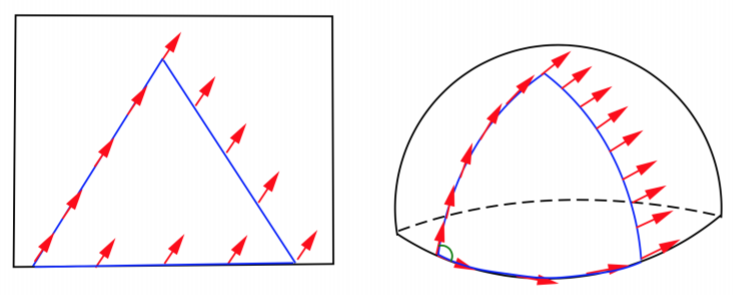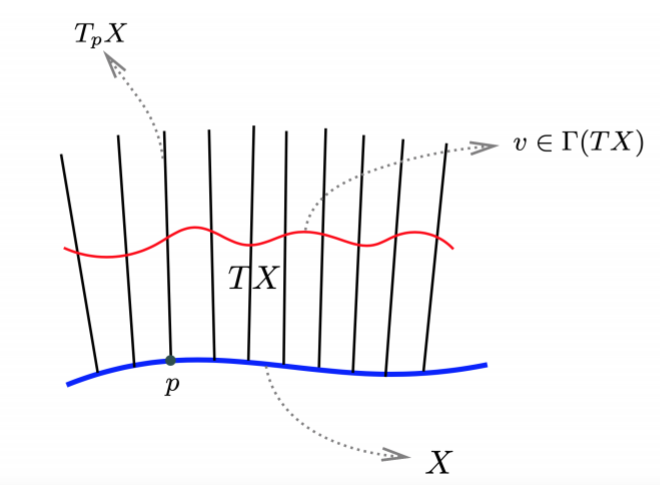## 联络Fig：平行移动示意图

$(\mathcal{L}_vu)^i = v^j\partial_ju^i - u^j\partial_j v^i$

### 协变微商

#### 协变微商

$X$$n$-维光滑流形，（仿射）联络 $\nabla$ 是一个映射，它将一个切矢量映为一个 $(1,1)$-型张量场，并满足

• $\nabla(u + v) =\nabla u + \nabla v$
• $\nabla (fv) = df \otimes v + f\nabla v$

$\nabla : \Gamma(TX) \rightarrow \Gamma(T^* X \otimes TX)$

$TX =\bigcup_ {p\in X}T_p X$

$\Gamma(TX) = \mathscr{X} (X)$• $T^*X$$TX$ 的对偶丛，可将其看成是 $\bigcup_{p\in X} T^*_pX$ 形成的集合并赋予了光滑结构。$T^*X$ 的截面是对偶矢量场或 $1$-形式场。
• $TX \otimes T^* X$ 称为切丛 $TX$ 和其对偶丛 $T^* X$ 的张量丛，其截面是流形 $X$ 上的光滑 $(1,1)$-型张量场。
• 用丛的语言来说，我们考虑的是切丛上的联络。按照习惯上，切丛 $TX$ 上的联络称为流形 $X$ 上的仿射联络（有时候也称为线性联络。线性联络和仿射联络往往可以混用，它们的区别和联系见 Kobayashi 的书）。

$u$ 是一个切矢量场，因 $\nabla v$ 是一个 $(1,1)$-型张量，因此可定义：

$\nabla _ u v = \nabla v(u)$

$\nabla_v$$(1,1)$-型张量，它作用在切矢量场 $u$ 后仍然得到一个切矢量场。从而 $\nabla_u$ 将一个切矢量场映成一个切矢量场，由定义很容易看出 $\nabla_u v$ 关于 $u$$\mathscr{F}(X)$-线性的，即我们有：

$\nabla_ {fu+gw} v = f\nabla_ u v + g\nabla_ w v$

$\nabla_ u v$ 称为切矢量场 $v$ 沿着切矢量场 $u$协变导数协变微商

$u = u^i \frac{\partial}{\partial x^i}, v = v^i \frac{\partial}{\partial x^i}$$u^i$$v^i$ 都是坐标域上的光滑函数。则我们有：

$\nabla v=\nabla (v^i\frac{\partial}{\partial x^i})=dv^i\otimes\frac{\partial}{\partial x^i}+v^i\nabla\frac{\partial}{\partial x^i}$

$\nabla(\frac{\partial}{\partial x_ i} )$ 是一个 $(1,1)$-型张量，因此局部上可以表示为：

$\nabla\frac{\partial}{\partial x^i}=\Gamma^k_{\ \ ji}dx^j\otimes\frac{\partial}{\partial x^k}=\omega^k_{\ \ i}\otimes \frac{\partial}{\partial x^k}$

$\omega^k_ {\ \ i} = \Gamma^k_{\ \ ji}dx^j$

$\nabla v=(\frac{\partial v^k}{\partial x^j}+\Gamma^k_{\ \ ji}v^i)dx^j\otimes\frac{\partial}{\partial x^k}$

$\nabla v=(dv^i+\omega^i_{\ \ j}v^j)\otimes\frac{\partial}{\partial x^k}$

\begin{aligned} \nabla_u v &= \nabla v(u) = (\frac{\partial v^k}{\partial x^j}+\Gamma^k_{\ \ ji}v^i)dx^j\otimes\frac{\partial}{\partial x^k}(u^l\frac{\partial}{\partial x^l})\\ &= u^j(\frac{\partial v^k}{\partial x^j}+\Gamma^k_{\ \ ji}v^i)\frac{\partial}{\partial x^k}\\ \end{aligned}\tag{1}

$\nabla_{\frac{\partial}{\partial x^j}}\frac{\partial}{\partial x^i}=\Gamma^k_{\ \ ji}\frac{\partial}{\partial x^k}$

$\nabla_{j}\frac{\partial}{\partial x^i}=\Gamma^k_{\ \ ji}\frac{\partial}{\partial x^k}$

#### 平行移动

$v(t)$ 是定义在曲线 $c : I \rightarrow X ,t \mapsto c(t)$ 上的切矢量。我们称 $v(t)$ 沿着曲线 $c$ 是平行移动的，若：

$\nabla_ u v = 0$

\begin{aligned} &u(t) = u^i(t)\frac{\partial}{\partial x^i} = \frac{dx^i(c(t))}{dt}\frac{\partial}{\partial x^i} = \frac{d(c(t))^i}{dt}\frac{\partial}{\partial x^i}\\ &v(t) = v^i(t)\frac{\partial}{\partial x^i} = v^i(c(t))\frac{\partial}{\partial x^i}\\ \end{aligned}

$\frac{dx^i(c(t))}{dt}\frac{\partial v^k}{\partial x^j} + \Gamma^k_{\ \ ji}v^i\frac{dx^j(c(t))}{dt} = 0$

$\frac{dv^k(t)}{dt}+\Gamma^k_{\ \ ji}u^j(t)v^i(t)=0$

#### 测地线

$\nabla_ u u = \lambda u$

$\nabla_ u u = 0$

$\frac{d^2 x^k}{dt^2}+\Gamma^k_{\ \ ji}\frac{dx^j}{dt}\frac{dx^i}{dt}=0$

### Koszul 联络

Koszul 在 1950 年代提出如下联络的定义，现在被广泛使用。

Koszul 联络
$u,v,w \in \Gamma(TX),\ f \in \mathscr{F}(X)$。联络 $\nabla$ 是一个映射：

$\nabla : \Gamma(TX)\times \Gamma(TX) \rightarrow \Gamma(TX)$

• $\nabla_u (v + w) = \nabla_ u v + \nabla_ u w$
• $\nabla_{fu} v = f\nabla_ u v$
• $\nabla_{u+v} w = \nabla_ u w + \nabla_ v w$
• $\nabla_ u (fv) = u(f)v + f\nabla_ u v$

### 联络的推广

#### 协变微商的推广

• 首先对于光滑函数 $f\in\mathscr{F}(X)$，我们可以定义 $\nabla f =df$。若将协变微商推广到一般张量，我们也期望 Leibniz 法则也能够适用。

• 其次，设 $v$ 是一个矢量场，而 $\theta$ 是一个对偶矢量场，则 $\theta(v) = \langle v, \theta\rangle = C(v\otimes \theta)$ 是一个光滑函数。我们可以将 $\nabla$ 扩张到对偶矢量场 $\theta$，使得下面表达式成立：

$\langle \nabla v,\theta\rangle + \langle v,\nabla\theta\rangle = \nabla\langle v,\theta\rangle = d\langle v,\theta\rangle$

\begin{aligned} d\langle v,\theta\rangle &= d\circ C(v\otimes \theta)\\ &= \nabla \circ C(v\otimes \theta)\\ \end{aligned}

\begin{aligned} \langle \nabla v,\theta\rangle + \langle v,\nabla\theta\rangle &= C(\nabla v\otimes \theta + v\otimes \nabla \theta)\\ &= C \circ \nabla (v\otimes \theta) \end{aligned}

$\nabla$ 是一个映射，它将 $(r,s)$-型张量场映为 $(r,s + 1)$-型张量场，并满足：

• $\nabla(\tau + \sigma) = \nabla\tau + \nabla\sigma$
• $\nabla f = df$
• $\nabla(\tau \otimes \phi) = \nabla\tau \otimes \phi + \tau \otimes \nabla\phi$
• $\nabla \circ C = C \circ \nabla$

$\langle \nabla e_i,e^{*j}\rangle + \langle e_i,\nabla e^{*j}\rangle = d\langle e_i,e^{*j}\rangle = 0$

$\nabla e_i = \omega^j_{\ \ i} \otimes e_j$

$\langle \omega^k_{\ \ i} \otimes e_k,e^{*j}\rangle + \langle e_i,\nabla e^{*j}\rangle = 0$

$\langle e_i,\nabla e^{*j}\rangle = -\omega^j_{\ \ i}$

$\nabla e^{*j} = \omega^{*j}_{\ \ \ \ k}\otimes e^{*k}$，其中 $\omega^{*j}_{\ \ \ \ k}$ 是一些 $1$-形式场，则有：

$\langle e_i,\omega^{*j}_{\ \ \ \ k}\otimes e^{*k}\rangle = \omega^{*j}_{\ \ \ \ i} = -\omega^j_{\ \ i}$

$\nabla \theta = \nabla(\theta_ie^{*i}) = (d\theta_i - \omega^j_{\ \ i}\theta_j)\otimes e^{*i}$

#### Koszul 联络的推广

• $\nabla_ u (\tau + \sigma) = \nabla_ u \tau + \nabla_u\sigma$
• $\nabla_ {fu} \tau = f\nabla_ u \tau$
• $\nabla_ {u+v} \tau = \nabla_ u \tau + \nabla_ v \tau$
• $\nabla_ u (\tau \otimes \phi) = \nabla_ u \tau \otimes \phi + \tau \otimes \nabla_ u \phi$
• $\nabla_ u f = u(f) = \mathcal{L}_ u f$
• $\nabla_ u \circ C = C \circ \nabla_ u$